TitleIntermediate Algebra
Answer/Discussion to Practice Problems
Tutorial 14: Graphing Linear EquationsAnswer/Discussion to 1a 2x - 3y = -6

 Let’s first find the x-intercept.  What value are we going to use for y?  You are correct if you said y = 0.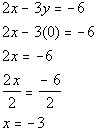*Find x-int. by replacing y with 0   *Inverse of mult. by 2 is div. by 2

 The x-intercept is (-3, 0).   Next we will find the y- intercept. What value are we going to plug in for x?  If you said x = 0, you are right.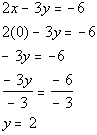*Find y-int. by replacing x with 0   *Inverse of mult. by -3 is div. by -3

 The y-intercept is (0, 2)

 We can plug in any x value we want as long as we get the right corresponding y value and the function exists there.  Let’s put in an easy number  x = 1: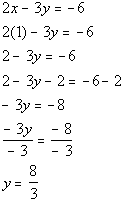*Replace x with 1   *Inverse of add 2 is sub. 2   *Inverse of mult. by -3 is div. by -3

So the ordered pair (1, 8/3) is another solution to our function.

Note that we could have plugged in any value for x: 5, 10, -25, ...,  but it is best to keep it as simple as possible.

The solutions that we found are:

 x y (x, y) -3 0 (-3, 0) 0 2 (0, 2) 1 8/3 (1, 8/3)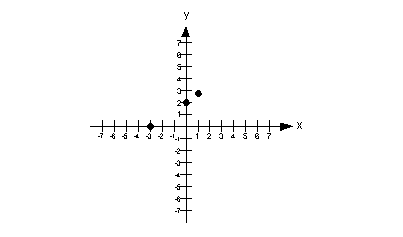Step 4:  Draw the graph.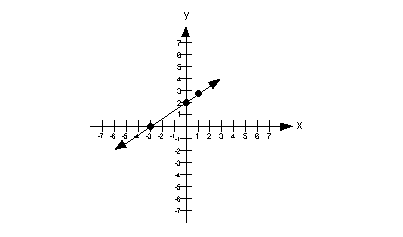(return to problem 1a)Answer/Discussion to 1b x = 3y

 Let’s first find the x-intercept.  What value are we going to use for y?  You are correct if you said y = 0.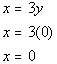*Find x-int. by replacing y with 0

 The x-intercept is (0, 0).   Next we will find the y- intercept. What value are we going to plug in for x?  If you said, x = 0 you are right.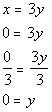*Find y-int. by replacing x with 0

 The y-intercept is (0, 0)

 Since we really have found only one point this time, we better find two  additional solutions so we have a total of three points. We can plug in any x value we want as long as we get the right corresponding y value and the function exists there.  Let’s put in an easy number  x = 1: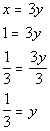*Replace x with 1 *Inverse of mult. by 3 is div. by 3

 So the ordered pair (1, 1/3) is another solution to our function.    Let’s put in another easy number x = -1: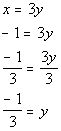*Replace x with -1 *Inverse of mult. by 3 is div. by 3

So the ordered pair (-1, -1/3) is another solution to our function.

The solutions that we found are:

 x y (x, y) 0 0 (0, 0) 1 1/3 (1, 1/3) -1 -1/3 (-1, -1/3)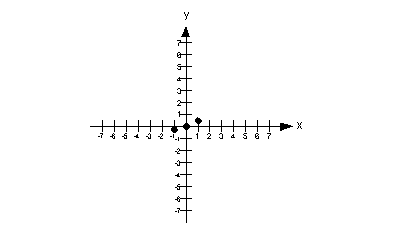Step 4:  Draw the graph.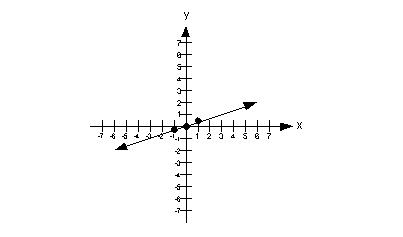(return to problem 1b)Answer/Discussion to 2a x = 4

 This is in the form x = c. So, what type of line are we going to end up with? Vertical.

Since this is a special type of line, I thought I would talk about steps 1 and 2 together.

It does not matter what y is, as long as x is 4.

Note that the x-intercept is at (4, 0).

Do we have a y-intercept?  The answer is no.  Since x can never equal 0, then there will be no y-intercept for this equation.

Some points that would be solutions are (4, 0), (4, 1), and (4, 2).

Again, I could have picked an infinite number of solutions.

The solutions that we found are:

 x y (x, y) 4 0 (4, 0) 4 1 (4, 1) 4 2 (4, 2 )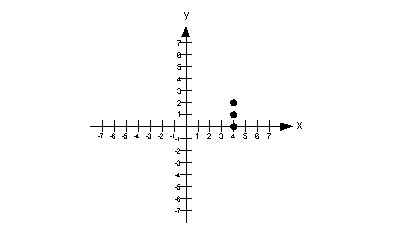Step 4:  Draw the graph.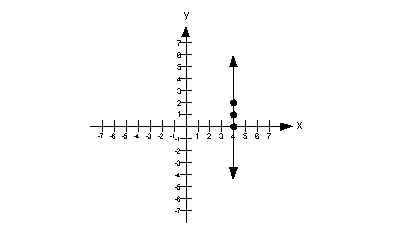(return to problem 2a)Answer/Discussion to 2b y + 5 = 0

 If you subtract 5 from both sides, you will have y = -5.  It looks like it fits the form y = c. With that in mind, what kind of line are we going to end up with? Horizontal.

Since this is a special type of line, I thought I would talk about steps 1 and 2 together.

It doesn’t matter what x is, y is always -5.  So for our solutions we just need three ordered pairs such that y = -5.

Note that the y-intercept (where x = 0) is at (0, -5).

Do we have a x-intercept? The answer is no.  Since y has to be -5, then it can never equal 0, which is the criteria of an x-intercept.

So some points that we can use are (0, -5), (1, -5) and (2, -5).  These are all ordered pairs that fit the criteria of y having to be -5.

Of course, we could have used other solutions, there are an infinite number of them.

The solutions that we found are:

 x y (x, y) 0 -5 (0, -5) 1 -5 (1, -5) -1 -5 (1, -5)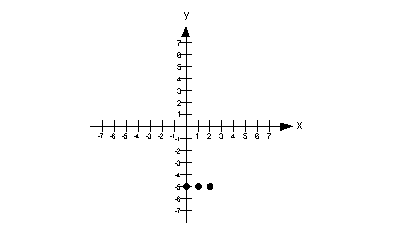Step 4:  Draw the graph.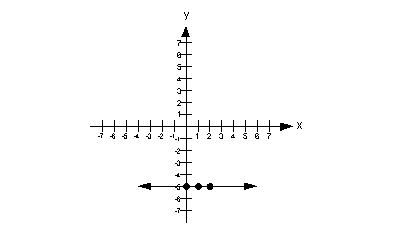(return to problem 2b)

Last revised on July 3, 2011 by Kim Seward.
All contents copyright (C) 2001 - 2011, WTAMU and Kim Seward. All rights reserved.